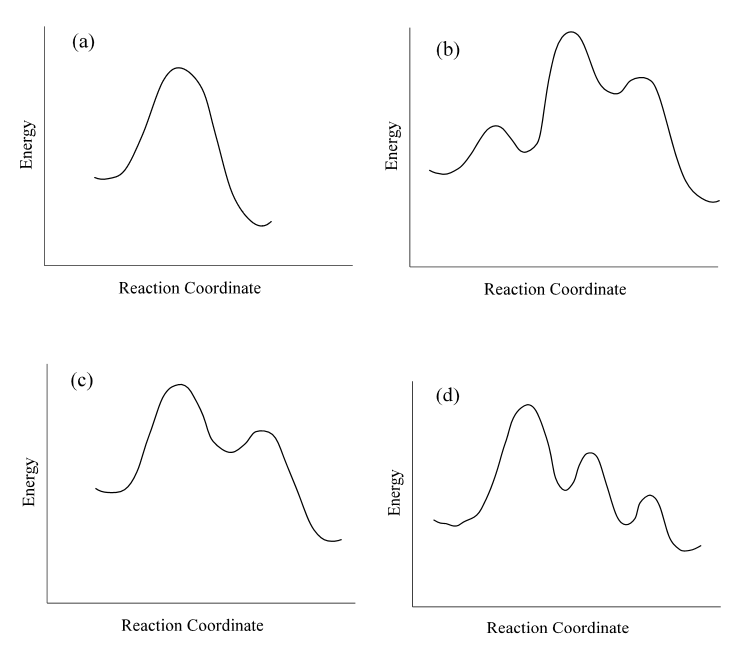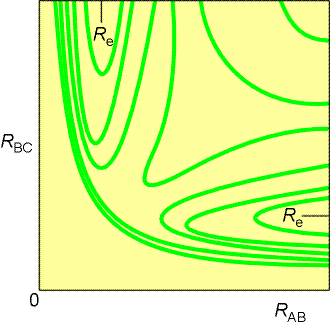# Homework 6 (Due 4/20/2016)

$$\newcommand{\vecs}{\overset { \rightharpoonup} {\mathbf{#1}} }$$ $$\newcommand{\vecd}{\overset{-\!-\!\rightharpoonup}{\vphantom{a}\smash {#1}}}$$$$\newcommand{\id}{\mathrm{id}}$$ $$\newcommand{\Span}{\mathrm{span}}$$ $$\newcommand{\kernel}{\mathrm{null}\,}$$ $$\newcommand{\range}{\mathrm{range}\,}$$ $$\newcommand{\RealPart}{\mathrm{Re}}$$ $$\newcommand{\ImaginaryPart}{\mathrm{Im}}$$ $$\newcommand{\Argument}{\mathrm{Arg}}$$ $$\newcommand{\norm}{\| #1 \|}$$ $$\newcommand{\inner}{\langle #1, #2 \rangle}$$ $$\newcommand{\Span}{\mathrm{span}}$$ $$\newcommand{\id}{\mathrm{id}}$$ $$\newcommand{\Span}{\mathrm{span}}$$ $$\newcommand{\kernel}{\mathrm{null}\,}$$ $$\newcommand{\range}{\mathrm{range}\,}$$ $$\newcommand{\RealPart}{\mathrm{Re}}$$ $$\newcommand{\ImaginaryPart}{\mathrm{Im}}$$ $$\newcommand{\Argument}{\mathrm{Arg}}$$ $$\newcommand{\norm}{\| #1 \|}$$ $$\newcommand{\inner}{\langle #1, #2 \rangle}$$ $$\newcommand{\Span}{\mathrm{span}}$$

Name: ______________________________

Section: _____________________________

Student ID#:__________________________

## Q6.1

Consider this acid-catalyzed iodine induced decomposition of hydrogen peroxide (unbalanced equation):

$H_2O_2 + 3 I^- \rightarrow H_2O + I_3^-$

The rate was that was experimentally determined to be:

$rate = k [H_2O_2][I^-][H^+]$

Which of the following mechanisms would be explain the measured rate law and balanced reaction?

Mechanism A:

$H_2O_2 + 3 I^- + 2H^+ \rightarrow 2H_2O + I_3^-$

Mechanism B:

$H^+ + I^- \rightleftharpoons HI \tag{fast equilibrium}$

$H_2O_2 + HI \rightarrow H_2O + HOI \tag{slow}$

$HOI+ I^- \rightarrow OH^- + I_2 \tag{fast}$

$OH^- + H^+ \rightarrow H_2O \tag{fast}$

$I^- +I_2 \rightarrow I_3^- \tag{fast}$

Mechanism C:

$H^+ + I^- \rightarrow HI \tag{slow}$

$H_2O_2 + HI \rightarrow H_2O + HOI \tag{fast}$

$HOI+ I^- \rightarrow OH^- + I_2 \tag{fast}$

$OH^- + H^+ \rightarrow H_2O \tag{fast}$

Mechanism D:

$H_2O_2 + H^+ + I^- \rightarrow H_2O + HOI \tag{slow}$

$HOI+ I^- \rightarrow OH^- + I_2 \tag{fast}$

$I^- +I_2 \rightarrow I_3^- \tag{fast}$

$OH^- + H^+ \rightarrow H_2O \tag{fast}$

## Q6.2

The reaction $H_{2(g)} + Br_{2(g)} \rightarrow 2HBr_{(g)}$ appears to occur view a multiple step mechanism:

$Br_{2} \rightleftharpoons 2 Br_{(g )} \tag{fast equilibrium}$

$H_{2(g)} + Br(g) \rightleftharpoons HBr_{(g)} + H_{(g)} \tag{slow}$

$H_{(g)} + Br_{(g)} \rightarrow HBr_{(g)} \tag{fast}$

1. What is the role of H(g) in the mechanism: reactant, product, reaction intermediate, or transition state?
2. If this mechanism is correct, what is the overall rate law for the reaction?
3. Which one of the following reaction diagrams best agrees with the mechanism? Identify all reactants, products, reaction intermediates, and transition states in the selected profile.## Q6.3

If the rate of a reaction is quadrupled when the temperature was increased from $$55^o$$ C to $$60^o$$ C. What is the activation barrier for this reaction?

## Q6.4

If the activation energy for a chemical reaction is 65.7 kJ/mol, then how many times faster will the reaction occur at $$50^o$$ C than $$0^o$$ C?

## Q6.5

This reaction is well studied to understand basic mechanisms of gas phase reaction dynamics: $H_{(g)} + H_{2(g)} \rightarrow H_{2(g)} + H_{(g)}$ A contour plot of the potential energy surface of the collinear collision of $$H$$ and $$H_2$$ is shown below, where $$r_{AB}$$ is the bond length of the reactant $$H_2$$ and $$r_{BC}$$ is the bond length of the product $$H_2$$ .

1. Label the transition state.
2. Draw a dashed line that indicates the lowest energy path of the reaction
3. What is the distance between the $$H$$ atom and each hydrogen of the $$H_2$$ atom in the transition state? (hint: A drawing of the transition state would probably help)## Q6.6

What two factors determine if a single collision will be productive in generating a product in collision theory?

## Q6.7

Cyclohexane converts between the chair” and “boat” conformations with the enthalpy and entropy of the formation of the activated complex are known to be 31.9 KJ mol-1 and 16.7 J K-1 mol-1, respectively.

1. Determine the Gibbs energy of activation and the rate constant for this conversion at room temperature.
2. Hypothesize what the structure of the transition state might be.

Homework 6 (Due 4/20/2016) is shared under a not declared license and was authored, remixed, and/or curated by LibreTexts.